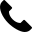+86-731-89578196[email protected]

Question: Are there any special procedures I need to follow before use sputtering target?

Answer: Most ceramic targets benefit from being bonded to a backing plate to increase target cooling. In addition, the target must be conditioned prior to depositing films from it.

Question: How do I find the mass or weight of a circular sputtering target (measurements in millimeters)?

Answer: Mass (m) = Pi x (D/20)2 x (T/10) x Density; where D = Diameter (millimeters), T = Thickness/Length (millimeters), Density (grams/cm3)

Question: How do I find the mass or weight of a circular sputtering target (measurements in inches)?

Answer: Mass (m) = Pi x (2.54D/2)2 x 2.54T x Density; where D = Diameter (inches), T = Thickness/Length (inches), Density (grams/cm3)

Question: How do I find the mass or weight of a rectangular sputtering target (millimeters)?

Answer: Mass (m) = L/10 x W/10 x T/10 x Density; where L = Length (millimeters), W = Width (millimeters), T = Thickness (millimeters), Density (grams/cm3)

Question: What types of sputtering targets do you recommend bonding to a backing plate?

Answer: Ceramic or inorganic compund targets because of fragility and poor heat transfer. Also, precious metal targets so a thinner target can be purchased.

Question: How do I clean oil-covered sputtering targets?

Answer: Sputtering targets that react with air or water vapor are protected during storage and shipment by immersion in a low viscosity (hydrocarbon) mineral oil. Before the material can be installed in a vacuum system, this oil must be completely removed using solvents that do not act as additional contamination sources for the vacuum system or the subsequent thin-film processes.